Essential Concept 99: The Full Fundamental Law | IFT World
101 Concepts for the Level I Exam

# Essential Concept 99: The Full Fundamental LawAccording to the full fundamental law, the expected active return is expressed as: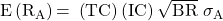Information ratio is expressed as: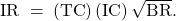The transfer coefficient is calculated as: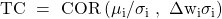It can take values from -1 to +1.

If there are no constraints and the actual portfolio optimal weights are equal to the actual weights, then TC will be equal to 1 and we will have the basic fundamental law.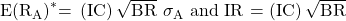The optimal amount of active risk in an actively managed portfolio with constraints is expressed as: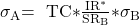The maximum value of the constrained portfolio’s Sharpe ratio is given as: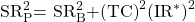Example: Consider an actively managed portfolio has a transfer coefficient of 0.50 and an unconstrained information ratio of 0.30. The benchmark portfolio has a Sharpe ratio of 0.40 and risk of 16.0%. What is the optimal amount of aggressiveness in the actively managed portfolio?

Solution: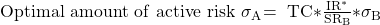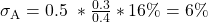If the actively managed portfolio is constructed with this amount of active risk, what is the Sharpe ratio?

Solution: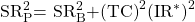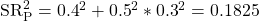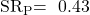If the constrained portfolio has an active risk of 8.0%, how can the active risk be lowered to the optimal level of 6.0%?

Solution:

The benchmark has a risk of 0% and the constrained portfolio has an active risk of 8.0%. To get an optimal level of 6.05, the weight of the actively managed fund must be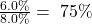. The actively managed portfolio will have an optimal risk of 6.0% if the weight in the benchmark is 25% and 75% in the actively managed fund.